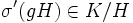# Characteristicity is quotient-transitive

This article gives the statement, and possibly proof, of a subgroup property (i.e., characteristic subgroup) satisfying a subgroup metaproperty (i.e., quotient-transitive subgroup)
View all subgroup metaproperty satisfactions | View all subgroup metaproperty dissatisfactions |Get help on looking up metaproperty (dis)satisfactions for subgroup properties
Get more facts about characteristic subgroup |Get facts that use property satisfaction of characteristic subgroup | Get facts that use property satisfaction of characteristic subgroup|Get more facts about quotient-transitive subgroup

## Statement

### Property-theoretic statement

The subgroup property of being a characteristic subgroup satisfies the subgroup metaproperty of being quotient-transitive.

### Statement with symbols

Suppose$H \le K \le G$ are subgroups such that$H$ is a characteristic subgroup of$G$, and$K/H$ is a characteristic subgroup of$G/H$. Then,$K$ is a characteristic subgroup of$G$.

## Proof

Given: A group$G$, subgroups$H \le K \le G$ such that$H$ is characteristic in$G$, and$K/H$ is characteristic in$G/H$

To prove:$K$ is characteristic in$G$

Proof: We pick any automorphism$\sigma$ of$G$, and want to show that$\sigma(K) = K$. For this, first observe that$\sigma(H) = H$, so$\sigma$ induces an automorphism on the quotient$G/H$, by the rule$gH \mapsto \sigma(g)H$. Call this automorphism$\sigma'$.

Then,$\sigma'$ is an automorphism of$G/H$. Since$K/H$ is characteristic in$G/H$,$\sigma'(K/H) = K/H$. Thus, for any$g \in K$,$\sigma'(gH) \in K/H$, and hence, unwrapping the definition,$\sigma(g) \in K$. Thus,$\sigma(K) \subset K$. Since the same holds for$\sigma^{-1}$, we conclude that$\sigma(K) = K$, completing the proof.